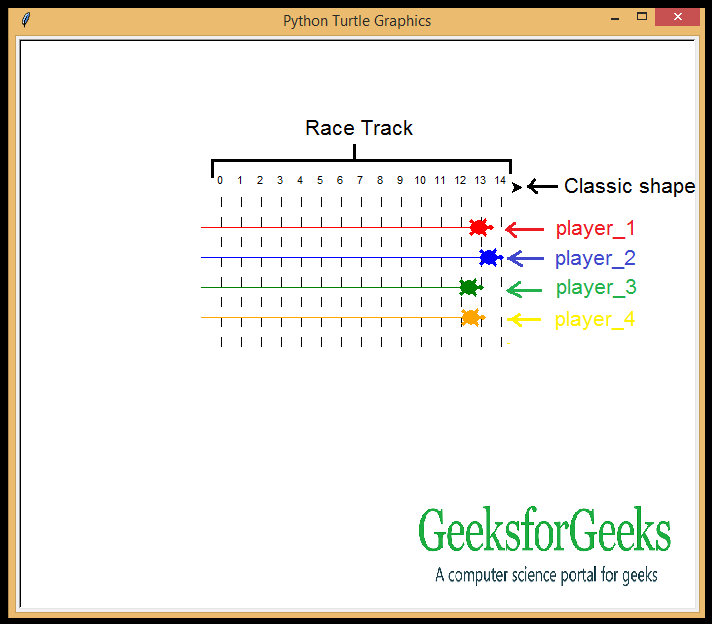# Create a simple Animation using Turtle in Python

Turtle is a Python feature like a drawing board, which lets us command a turtle to draw all over it! We can use functions like turtle.forward(…) and turtle.right(…) which can move the turtle around. Let’s create a basic animation where different little turtles race around a track created for them.

Prerequisites: Turtle Programming in Python

### Requirements:

• Turtle Module
• Random Module

### Approach:

• Firstly, import the required modules.
• There are different shapes of pointer available in the turtle module(like arrow, classic and turtle). As this is a “Turtle Race”, we need the ‘turtle’ and ‘classic’ shape.
• We’ll use the classic shape to draw the racing track.
• List the specs of the first turtle, enter the turtle in the track and set the turtle ready for the race.
• Do the same for the rest of the turtles.
• Use the randint() to set the speed of the turtles.

Below is the implementation.

## Python3

 `# required modules` `from` `turtle ``import` `*` `from` `random ``import` `randint` ` `  `    `  `# classic shape turtle` `speed(``0``)` `penup()` `goto(``-``140``, ``140``)` ` `  `# racing track`   `for` `step ``in` `range``(``15``):` `    ``write(step, align ``=``'center'``)` `    ``right(``90``)` `    `  `    ``for` `num ``in` `range``(``8``):` `        ``penup()` `        ``forward(``10``)` `        ``pendown()` `        ``forward(``10``)` `        `  `    ``penup()` `    ``backward(``160``)` `    ``left(``90``)` `    ``forward(``20``)`   `# first player details` `player_1 ``=` `Turtle()` `player_1.color(``'red'``)` `player_1.shape(``'turtle'``)` ` `  `# first player proceeds to racing track` `player_1.penup()` `player_1.goto(``-``160``, ``100``)` `player_1.pendown()` ` `  `# 360 degree turn` `for` `turn ``in` `range``(``10``):` `    ``player_1.right(``36``)`   `# second player details` `player_2 ``=` `Turtle()` `player_2.color(``'blue'``)` `player_2.shape(``'turtle'``)` ` `  `# second player enters in the racing track` `player_2.penup()` `player_2.goto(``-``160``, ``70``)` `player_2.pendown()` ` `  `# 360 degree turn` `for` `turn ``in` `range``(``72``):` `    ``player_2.left(``5``)`   `# third player details` `player_3 ``=` `Turtle()` `player_3.shape(``'turtle'``)` `player_3.color(``'green'``)` ` `  `# third player enters in the racing track` `player_3.penup()` `player_3.goto(``-``160``, ``40``)` `player_3.pendown()` ` `  `# 360 degree turn` `for` `turn ``in` `range``(``60``):` `    ``player_3.right(``6``)`   `# fourth player details` `player_4 ``=` `Turtle()` `player_4.shape(``'turtle'``)` `player_4.color(``'orange'``)` ` `  `# fourth player enters in the racing track` `player_4.penup()` `player_4.goto(``-``160``, ``10``)` `player_4.pendown()` ` `  `# 360 degree turn` `for` `turn ``in` `range``(``30``):` `    ``player_4.left(``12``)`   `# turtles run at random speeds` `for` `turn ``in` `range``(``100``):` `    ``player_1.forward(randint(``1``, ``5``))` `    ``player_2.forward(randint(``1``, ``5``))` `    ``player_3.forward(randint(``1``, ``5``))` `    ``player_4.forward(randint(``1``, ``5``))`

Output:Output Explanation:

1. The code starts by creating a new turtle object and setting its color to red.
2. The turtle’s shape is set to the classic shape of a turtle, which is (0, 0), (140, 140), and (-140, 140).
3. Next, the code creates a speed variable and sets it to zero.
4. The penup() function is then called, which causes the turtle to stand up straight.
5. Next, the goto function is used to move the turtle 140 pixels towards the right side of the screen.
6. The for loop starts at step 15 and runs for 8 steps.
7. At each step, four different actions are performed: first, write(step) displays text in centered position; second, right(90) moves the cursor 90 pixels to the right; thirdly forward(10) moves the turtle 10 pixels forward; fourthly pendown() pauses execution for a moment so that drawing can be resumed with next line.
8. Finally at step 16 forward(20) is executed moving the turtle 20 pixels further forward on screen.
9. At step 17 player_1 = Turtle() assigns player_1 as an instance variable of player_1 object created at previous step.
10. This object has two properties: color and shape .
11. Color property holds value
12. The code creates a Turtle object and sets its color to red.
13. It also sets the shape of the turtle to be that of a classic turtle.
14. The code then starts a loop that will run for 15 steps.
15. At each step, the code will write out the value of step in centered text.
16. After writing out the step, the code will move right 90 degrees and then do a series of eight random math operations with num as their parameter.
17. These operations will result in the following: -Pen up -Forward 10 pixels -Pendown -Forward 10 pixels -Pen up -Backward 160 pixels -Left 90 degrees After completing these eight random operations, the code will once again.

Whether you're preparing for your first job interview or aiming to upskill in this ever-evolving tech landscape, GeeksforGeeks Courses are your key to success. We provide top-quality content at affordable prices, all geared towards accelerating your growth in a time-bound manner. Join the millions we've already empowered, and we're here to do the same for you. Don't miss out - check it out now!

Previous
Next